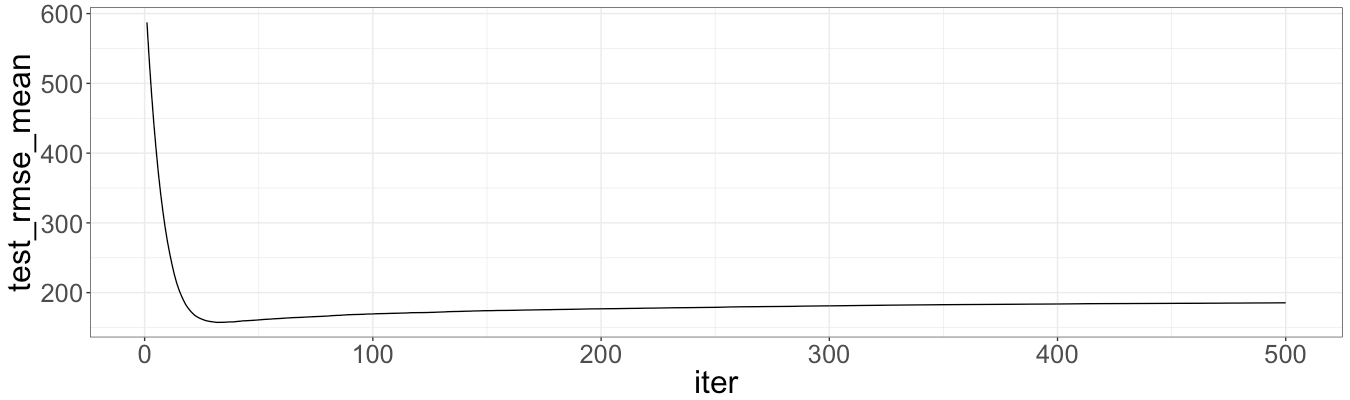Want to share your content on R-bloggers? click here if you have a blog, or here if you don't.

In pervious posts traditional regression models were fitted to real estate data. In this post tree-based models, namely random forests and gradient boosting, are trained to predict prices of the rent. These methods typically outperform traditional regression models yielding smaller errors. Furthermore, tree-based methods are much more robust to overfitting, which makes them superior in terms of prediction. However, the main disadvantage (and the reason why there is no love in insurance industry) is difficulties with interpretability.

## Random forests

Originally random forests are implemented in `randomForest` package. There is also a faster implementation in `ranger` package, which is used further. As usual, we start with some preliminary code to clean out the memory, load packages and data.

```packages <- c("ggplot2", "magrittr", "vtreat", "ranger", "xgboost", "caret")
sapply(packages, library, character.only = TRUE, logical.return = TRUE)

theme_set(theme_bw())
theme_update(text = element_text(size = 24))

rm(list = ls())

setwd("/Users/irudnyts/Documents/data/")
set.seed(1)
```

For random forests there is a hyperparameter `mtry` determining the number of columns to split in each node. As long as we have only two columns, we set `mtry = 2` (the model with default `mtry = 1` yields higher RMSE). We also use default number of trees (500 trees). The main function to run the regression is `ranger`. The function `predict`(`.ranger`) behaves a bit differently from other methods. Instead of returning a vector, it returns a list of vectors of class `ranger.prediction`. Thus, we need to extract `predictions` form that class.

```rf <- ranger(formula = price ~ area + rooms, data = property, mtry = 2)
predicted <- predict(rf, property)
(predicted\$predictions - property\$price) ^ 2 %>% mean() %>% sqrt()
#  93.07542
```

Random forests model outperform all traditional regression models in previous posts in terms of in-sample RMSE. Let’s now cross-validate model by looking at out-of-sample RMSE:

```property\$pred_rf <- NULL

folds <- kWayCrossValidation(nRows = nrow(property), nSplits = 3)

for(fold in folds) {
rf <- ranger(formula = price ~ area + rooms,
data = property[fold\$train, ],
mtry = 2)
property[fold\$app, "pred_rf"] <-
predict(rf, property[fold\$app, ])\$predictions
}

(property\$price - property\$pred_rf) ^ 2 %>% mean() %>% sqrt()
#  152.3637
```

The out-of-sample RMSE is comparable with one returned by linear model, but larger than for GAM IG.

This methods iteratively optimize the RMSE (or other accuracy measure) on training data. Thus, gradient boosting is more exposed to overfit. In order to get the optimal ‘nrounds’ (the maximum number of iterations) we need to use cross-validation technique, implemented in `xgb.cv`, which also calculates out-of-sample RMSE. The number of folds we keep equals to \$3\$, to be consistent with pervious analysis. The learning rate parameter is set to be \$0.1\$, a little bit less than default (\$0.3\$), implying robustness to overfit, but also slower speed.

```xg <- xgb.cv(data = as.matrix(property[, 2:3]),
label = property[, 1],
nfold = 3,
nrounds = 500,
metrics = "rmse",
eta = 0.1)
which.min(xg\$evaluation_log\$test_rmse_mean)
#  32
ggplot(data = xg\$evaluation_log) + geom_line(aes(x = iter, y = test_rmse_mean))
```The minimum out-of-sample RMSE is showed by the model with `nrounds = 32`. This value is approximate and depends on a seed. We use `nrounds = 40`. Let’s calculate RMSE for the optimal model and then out-of sample RMSE:

```xg <- xgboost(data = as.matrix(property[, 2:3]),
label = property[, 1],
nfold = 3,
nrounds = 34,
eta = 0.1)

property\$pred_xg <- predict(xg, as.matrix(property[, 2:3]))

(property\$price - property\$pred_xg) ^ 2 %>% mean() %>% sqrt()
#  104.8407

property\$pred_xg <- NULL
set.seed(1)
folds <- kWayCrossValidation(nRows = nrow(property), nSplits = 3)

for(fold in folds) {
xg <- xgboost(data = as.matrix(property[fold\$train, 2:3]),
label = property[fold\$train, 1],
nfold = 3,
nrounds = 40,
eta = 0.1)
property[fold\$app, "pred_xg"] <-
predict(xg, as.matrix(property[fold\$app, 2:3]))
}

(property\$price - property\$pred_xg) ^ 2 %>% mean() %>% sqrt()
#  145.9076
```

Unfortunately, XGboost model does not achieve smaller RMSE than previous models.

As summary, tree based methods are not always better solution for prediction. As we see, the out-of-sample RMSE is similar to one for linear model. At the same time, tree-based methods lose precious interpretability.

Note: Both packages have built-in cross-validation functions. However, for proper comparison one has to train models on the same training data set applying, then, to the same test set.